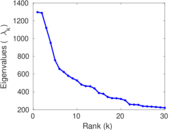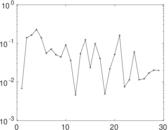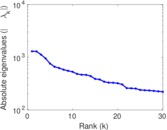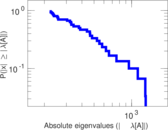# Wikipedia edits (zh-yue)

This is the bipartite edit network of the Cantonese Wikipedia. It contains users and pages from the Cantonese Wikipedia, connected by edit events. Each edge represents an edit. The dataset includes the timestamp of each edit.

 Code `zh-yue` Internal name `edit-zh_yuewiki` Name Wikipedia edits (zh-yue) Data source http://dumps.wikimedia.org/ AvailabilityDataset is available for download Consistency checkDataset passed all tests Category Authorship network Dataset timestamp 2017-10-20 Node meaning User, article Edge meaning Edit Network formatBipartite, undirected Edge typeUnweighted, multiple edges Temporal dataEdges are annotated with timestamps

## Statistics

 Size n = 160,909 Left size n1 = 10,433 Right size n2 = 150,476 Volume m = 947,552 Unique edge count m̿ = 473,904 Wedge count s = 2,105,881,124 Claw count z = 13,892,847,708,665 Cross count x = 91,067,965,048,299,552 Square count q = 3,041,045,418 4-Tour count T4 = 32,752,994,956 Maximum degree dmax = 58,016 Maximum left degree d1max = 58,016 Maximum right degree d2max = 1,581 Average degree d = 11.777 5 Average left degree d1 = 90.822 6 Average right degree d2 = 6.297 03 Fill p = 0.000 301 866 Average edge multiplicity m̃ = 1.999 46 Size of LCC N = 153,487 Diameter δ = 13 50-Percentile effective diameter δ0.5 = 3.505 37 90-Percentile effective diameter δ0.9 = 5.207 56 Median distance δM = 4 Mean distance δm = 4.040 10 Gini coefficient G = 0.855 344 Balanced inequality ratio P = 0.138 977 Left balanced inequality ratio P1 = 0.052 339 1 Right balanced inequality ratio P2 = 0.193 332 Power law exponent γ = 2.698 48 Tail power law exponent γt = 1.981 00 Tail power law exponent with p γ3 = 1.981 00 p-value p = 0.000 00 Left tail power law exponent with p γ3,1 = 1.691 00 Left p-value p1 = 0.077 000 0 Right tail power law exponent with p γ3,2 = 2.001 00 Right p-value p2 = 0.000 00 Degree assortativity ρ = −0.139 840 Degree assortativity p-value pρ = 0.000 00 Spectral norm α = 1,296.30 Algebraic connectivity a = 0.006 260 89 Spectral separation |λ1[A] / λ2[A]| = 1.006 82 Controllability C = 139,814 Relative controllability Cr = 0.887 049

## Plots

### Fruchterman–Reingold graph drawing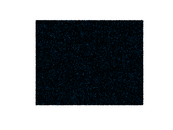### Degree distribution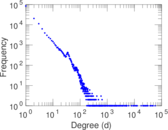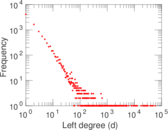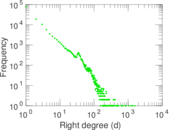### Cumulative degree distribution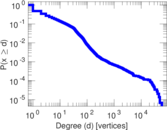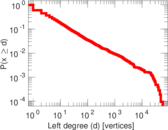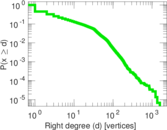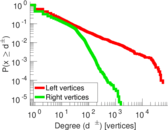### Lorenz curve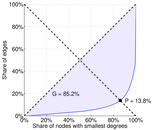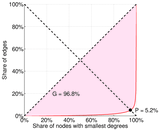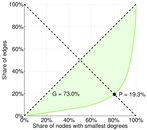### Spectral distribution of the adjacency matrix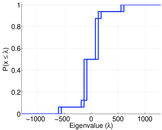### Spectral distribution of the normalized adjacency matrix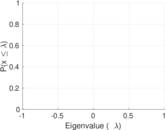### Spectral distribution of the Laplacian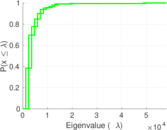### Spectral graph drawing based on the adjacency matrix### Spectral graph drawing based on the Laplacian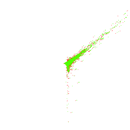### Spectral graph drawing based on the normalized adjacency matrix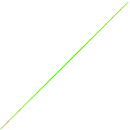### Degree assortativity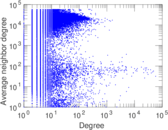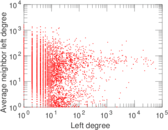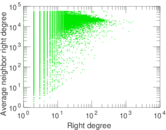### Zipf plot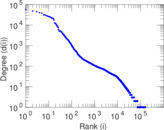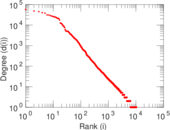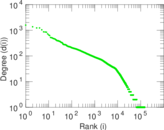### Hop distribution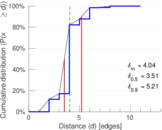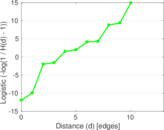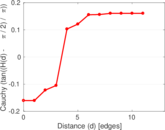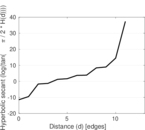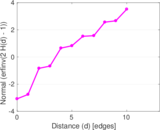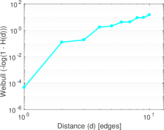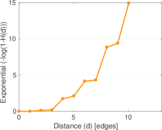### Edge weight/multiplicity distribution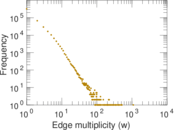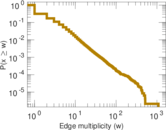### Temporal distribution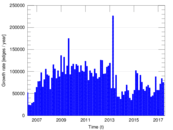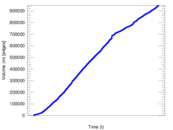### Diameter/density evolution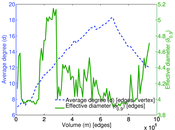### Matrix decompositions plots